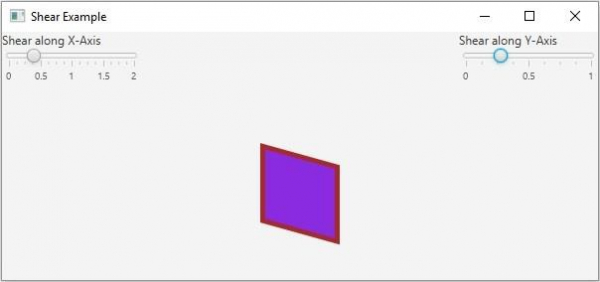# What is shear transform in JavaFX?

Displacement of an object in a fixed direction such that its shape is slanted is known as shear transform. This is also known as skewing.

In JavaFX using the object of the javafx.scene.transform.Shear class, you can skew a node along the required axis. Internally this class rotates the specified axis such that X and Y axes are no longer perpendicular.

This class contains four properties −

• The pivotX property (double) specifying the x coordinates of the shear pivot point. You can set the value to this property using the setPivotX() method.

• The pivotY property (double) specifying the y coordinates of the shear pivot point. You can set the value to this property using the setPivotY() method.

• The x property (double) specifying the shear x. You can set the value to this property using the setX() method.

• The x property (double) specifying the shear y. You can set the value to this property using the setY() method.

Every node in JavaFX contains an observable list to hold all the transforms to be applied on a node. You can get this list using the getTransforms() method.

To skew a Node −

• Instantiate the Shear class.

• Set the shear x (or, shear y) and pivot points (optional) using the respective setter methods.

• Get the list of transforms from the node (which you want to move) using the getTransforms() method.

• Add the above-created shear object to it.

• Add the node to the scene.

## Example

Following the JavaFX, example demonstrates the shear transform. It contains a 2D geometric shape and two sliders, representing the x and y skew values. If you move the sliders the object skies the chosen axis.

import javafx.application.Application;
import javafx.beans.value.ChangeListener;
import javafx.beans.value.ObservableValue;
import javafx.scene.Scene;
import javafx.scene.control.Label;
import javafx.scene.control.Slider;
import javafx.scene.layout.BorderPane;
import javafx.scene.layout.VBox;
import javafx.scene.paint.Color;
import javafx.scene.shape.Rectangle;
import javafx.scene.transform.Shear;
import javafx.stage.Stage;
public class ShearExample extends Application {
public void start(Stage stage) {
//Creating a rectangle
Rectangle rect = new Rectangle(300, 100, 75, 75);
rect.setFill(Color.BLUEVIOLET);
rect.setStrokeWidth(5.0);
rect.setStroke(Color.BROWN);
//Setting the slider for the horizontal translation
Slider slider1 = new Slider(0, 2, 0);
slider1.setShowTickLabels(true);
slider1.setShowTickMarks(true);
slider1.setMajorTickUnit(0.5);
slider1.setBlockIncrement(0.3);
//Creating shear transformation
Shear shear = new Shear();
//Setting the pivot points
shear.setPivotX(200);
shear.setPivotY(250);
//Setting the dimensions for the shear
public void changed(ObservableValue <?extends Number>observable, Number oldValue, Number newValue){
shear.setX((double) newValue);
shear.setY(0.0);
}
});
//Setting the slider for the vertical translation
Slider slider2 = new Slider(0, 1, 0);
slider2.setShowTickLabels(true);
slider2.setShowTickMarks(true);
slider2.setMajorTickUnit(0.5);
slider2.setBlockIncrement(0.3);
//Creating the translation transformation
public void changed(ObservableValue <?extends Number>observable, Number oldValue, Number newValue){
shear.setX(0.0);
shear.setY((double) newValue);
}
});
//Adding the transformation to the sphere
//Creating the pane
BorderPane pane = new BorderPane();
pane.setLeft(new VBox(new Label("Shear along X-Axis"), slider1));
pane.setRight(new VBox(new Label("Shear along Y-Axis"), slider2));
pane.setCenter(rect);
//Preparing the scene
Scene scene = new Scene(pane, 600, 250);
stage.setTitle("Shear Example");
stage.setScene(scene);
stage.show();
}
public static void main(String args[]){
launch(args);
}
}

## Output